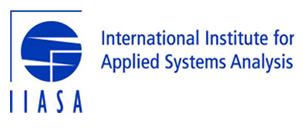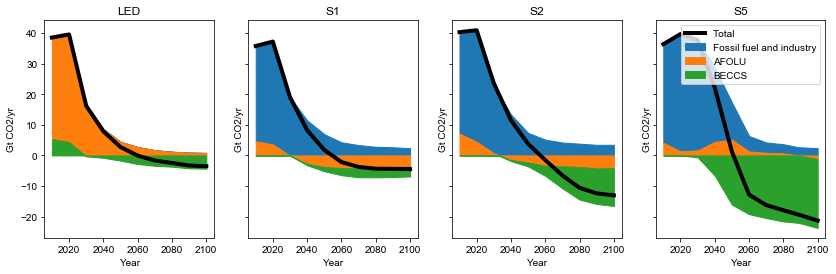# Figures and indicators for SPM Figure 3b of illustrative pathways¶

## Notebook spm_sr15_figure_3b_illustrative_pathways¶

This notebook is based on the Release 1.1 of the IAMC 1.5C Scenario Explorer and Data and refers to the published version of the IPCC Special Report on Global Warming of 1.5C (SR15).

The notebook is run with pyam release 0.5.0.

The source code of this notebook is available on GitHub (release 2.0.2).

spm_sr15_figure_3b_illustrative_pathways

### IPCC SR15 scenario assessment¶# Characteristics of four illustrative model pathways¶

## Figure 3b of the Summary for Policymakers¶

This notebook derives the figure panels and indicators for the table in Figure 3b in the Summary for Policymakers of the IPCC's "Special Report on Global Warming of 1.5°C".

The scenario data used in this analysis can be accessed and downloaded at https://data.ene.iiasa.ac.at/iamc-1.5c-explorer.

## Load pyam package and other dependencies¶

In :
import pandas as pd
import numpy as np
import io
import itertools
import yaml
import math
import matplotlib.pyplot as plt
plt.style.use('style_sr15.mplstyle')
%matplotlib inline
import pyam

pyam - INFO: Running in a notebook, setting pyam logging level to logging.INFO and adding stderr handler


## Import scenario data, categorization and specifications files¶

Alternatively, it can be re-created using the notebook sr15_2.0_categories_indicators.

The last cell of this section loads and assigns a number of auxiliary lists as defined in the categorization notebook.

In :
sr1p5 = pyam.IamDataFrame(data='../data/iamc15_scenario_data_world_r2.0.xlsx')

pyam.utils - INFO: Reading ../data/iamc15_scenario_data_world_r2.0.xlsx

In :
sr1p5.load_meta('sr15_metadata_indicators.xlsx')

pyam.core - INFO: Importing metadata for 416 scenarios (for total of 416)

In :
with open("sr15_specs.yaml", 'r') as stream:

rc = pyam.run_control()
for item in specs.pop('run_control').items():
rc.update({item: item})
cats_15 = specs.pop('cats_15')
cats_15_no_lo = specs.pop('cats_15_no_lo')
marker = specs.pop('marker')


## Downselect scenario ensemble to categories of interest for this assessment¶

In :
sr1p5.meta.rename(columns={'Kyoto-GHG|2010 (SAR)': 'kyoto_ghg_2010'}, inplace=True)

In :
df = sr1p5.filter(category=cats_15)


## Global carbon dioxide emissions in four illustrative pathways¶

Figure SPM3b shows the contribution to CO2 emissions and removal by three categories in the four illustrative pathways.

This illustration does not use the emissions timeseries as reported by the models. This is because the variable Emissions|CO2|Energy and Industrial Processes represents net emissions, incorporating carbon dioxide removal in this sector.

The steps below compute the gross emissions. The long variable names are mapped to short variables for easier readibility.

In :
afolu_var = 'Emissions|CO2|AFOLU'
ene_ind_var = 'Emissions|CO2|Energy and Industrial Processes'
beccs_var ='Carbon Sequestration|CCS|Biomass'


We downselect the entire data to the four illustrative pathways (marker scenarios) and the three variables of interest. For consistency with the figure in the SPM, the units are converted to Gt CO2.

In :
pw = df.filter(marker=marker, variable=[afolu_var, ene_ind_var, beccs_var],
year=range(2010, 2101, 10))

In :
pw.convert_unit('Mt CO2/yr', 'Gt CO2/yr', inplace=True)


As a first step, we extract the timeseries for the AFOLU emissions and rename the variable for brevity. This data will be used as is in this figure.

In :
afolu = (
pw.filter(variable=afolu_var)
.rename(variable={afolu_var: 'AFOLU'})
)


The energy-and-industry and BECCS timeseries data needs some processing. It is first separated into two distinct dataframes, and the BECCS variable is renamed for brevity.

In :
ene_ind = pw.filter(variable=ene_ind_var)

In :
beccs = (
pw.filter(variable=beccs_var)
.rename(variable={beccs_var: 'BECCS'})
)


The variable Carbon Sequestration|CCS|Biomass reports removed carbon dioxide as positive values. For use in this figure, the sign needs to be reversed.

In :
beccs.data.value = - beccs.data.value


The LED marker scenario does not use any BECCS by assumption of the scenario design. For this reason, the variable Carbon Sequestration|CCS|Biomass was not defined when the MESSAGE team submitted the scenario results to the IAMC 1.5°C Scenario Data ensemble.

For easier computation, we add this data series manually here.

In :
years = beccs.timeseries().columns

In :
beccs.append(
pyam.IamDataFrame(
pd.DataFrame( * len(years), index=years).T,
model='MESSAGEix-GLOBIOM 1.0', scenario='LowEnergyDemand',
region='World', variable='BECCS', unit='Gt CO2/yr'),
inplace=True
)


As a third step, we compute the difference between net CO2 emissions from the energy sector & industry and BECCS to obtain gross CO2 emissions in that sector.

In :
def get_value(df):
cols = ['model', 'scenario', 'region', 'year', 'unit']
return df.data.set_index(cols)['value']

In :
diff = get_value(ene_ind) - get_value(beccs)

In :
ene_ind_gross = pyam.IamDataFrame(diff, variable='Fossil fuel and industry')


We now combine the three contribution dataframes into one joint dataframe for plotting. Because the beccs IamDataFrame was partially altered, concatenating directly causes an issue, so we remove all meta columns from that dataframe beforehand.

In :
beccs.meta = beccs.meta.drop(columns=beccs.meta.columns)

In :
co2 = pyam.concat([ene_ind_gross, afolu, beccs])


We now proceed to plot the four illustrative pathways.

In :
fig, ax = plt.subplots(1, 4, figsize=(14, 4), sharey=True)

for i, m in enumerate(['LED', 'S1', 'S2', 'S5']):
co2.filter(marker=m).stack_plot(ax=ax[i], total=True, legend=False)
ax[i].title.set_text(m)

ax.legend(loc=1)

Out:
<matplotlib.legend.Legend at 0x1a25eeca50>## Collecting indicators across illustrative pathways¶

### Initialize a pyam.Statistics instance¶

In :
base_year = 2010
compare_years = [2030, 2050]
years = [base_year] + compare_years

In :
stats = pyam.Statistics(df=df, groupby={'marker': ['LED', 'S1', 'S2', 'S5']},
filters=[(('pathways', 'no & lo os 1.5'), {'category': cats_15_no_lo})])


### CO2 and Kyoto GHG emissions reductions¶

In :
co2 = (
df.filter(kyoto_ghg_2010='in range', variable='Emissions|CO2', year=years)
.convert_unit('Mt CO2/yr', 'Gt CO2/yr')
.timeseries()
)

In :
for y in compare_years:
stats.add((co2[y] / co2 - 1) * 100,
'CO2 emission reduction (% relative to 2010)',

In :
kyoto_ghg = (
df.filter(kyoto_ghg_2010='in range', variable='Emissions|Kyoto Gases (SAR-GWP100)', year=years)
.rename(unit={'Mt CO2-equiv/yr': 'Mt CO2e/yr'})
.convert_unit('Mt CO2e/yr','Gt CO2e/yr')
.timeseries()
)
for y in compare_years:
stats.add((kyoto_ghg[y] / kyoto_ghg[base_year] - 1) * 100,
'Kyoto-GHG emission reduction (SAR-GWP100), % relative to {})'.format(base_year),


### Final energy demand reduction relative to 2010¶

In :
fe = df.filter(variable='Final Energy', year=years).timeseries()
for y in compare_years:
stats.add((fe[y] / fe[base_year] - 1) * 100,
'Final energy demand reduction relative to {} (%)'.format(base_year),


### Share of renewables in electricity generation¶

In :
def add_stats_share(stats, var_list, name, total, total_name, years, df=df):

_df = df.filter(variable=var_list)
for v in var_list:
_df.require_variable(v, exclude_on_fail=True)
_df.filter(exclude=False, inplace=True)

component = (
_df.timeseries()
.groupby(['model', 'scenario']).sum()
)
share = component / total * 100

for y in years:

In :
ele = df.filter(variable='Secondary Energy|Electricity', year=compare_years).timeseries()
ele.index = ele.index.droplevel([2, 3, 4])

In :
ele_re_vars = [
'Secondary Energy|Electricity|Biomass',
'Secondary Energy|Electricity|Non-Biomass Renewables'
]
add_stats_share(stats, ele_re_vars, 'renewables', ele, 'electricity', compare_years)

pyam.core - INFO: All scenarios have the required variable Secondary Energy|Electricity|Biomass
pyam.core - INFO: All scenarios have the required variable Secondary Energy|Electricity|Non-Biomass Renewables


### Changes in primary energy mix¶

In :
mapping = [
('coal', 'Coal'),
('oil', 'Oil'),
('gas', 'Gas'),
('nuclear', 'Nuclear'),
('bioenergy', 'Biomass'),
('non-biomass renewables', 'Non-Biomass Renewables')
]

In :
for (n, v) in mapping:
data = df.filter(variable='Primary Energy|{}'.format(v), year=years).timeseries()

for y in compare_years:
stats.add((data[y] / data[base_year] - 1) * 100,
header='Primary energy from {} (% rel to {})'.format(n, base_year),


### Cumulative carbon capture and sequestration until the end of the century¶

In :
def cumulative_ccs(variable, name, first_year=2016, last_year=2100):

data = (
df.filter(variable=variable)
.convert_unit('Mt CO2/yr', 'Gt CO2/yr')
.timeseries()
)

data.apply(pyam.cumulative, raw=False, axis=1,
first_year=first_year, last_year=last_year),

In :
cumulative_ccs('Carbon Sequestration|CCS', 'CCS')

In :
cumulative_ccs('Carbon Sequestration|CCS|Biomass', 'BECCS')


### Land cover for energy crops¶

Convert unit to SI unit (million square kilometers).

In :
energy_crops = (
df.filter(variable='Land Cover|Cropland|Energy Crops', year=2050)
.convert_unit('million ha', 'million km2', factor=0.01)
.timeseries()
)

In :
stats.add(energy_crops, header='Land are for energy crops (million km2)')


### Emissions from land use¶

In :
species = ['CH4', 'N2O']

In :
for n in species:
data = df.filter(kyoto_ghg_2010='in range', variable='Emissions|{}|AFOLU'.format(n), year=years).timeseries()

for y in compare_years:
stats.add((data[y] / data[base_year] - 1) * 100,
header='Agricultural {} emissions (% rel to {})'.format(n, base_year),


## Display summary statistics and export to xlsx¶

In :
summary = stats.summarize(interquartile=True, custom_format='{:.0f}').T
summary

Out:
marker pathways
LED S1 S2 S5 no & lo os 1.5
mean (interquartile range)
count 52
CO2 emission reduction (% relative to 2010) 2030 -58 -47 -41 4 -49 (-40, -58) 
2050 -93 -95 -91 -97 -101 (-94, -107) 
Kyoto-GHG emission reduction (SAR-GWP100), % relative to 2010) 2030 -50 -49 -35 -2 -45 (-39, -51) 
2050 -82 -89 -78 -80 -87 (-81, -93) 
Final energy demand reduction relative to 2010 (%) 2030 -15 -5 17 39 -2 (7, -12) 
2050 -32 2 21 44 8 (22, -11) 
Share of renewables in electricity (%) 2030 60 58 48 25 56 (65, 47) 
2050 77 81 63 70 78 (86, 69) 
Primary energy from coal (% rel to 2010) 2030 -78 -61 -75 -59 -67 (-59, -78) 
2050 -97 -77 -73 -97 -82 (-74, -95) 
Primary energy from oil (% rel to 2010) 2030 -37 -13 -3 86 -15 (3, -34) 
2050 -87 -50 -81 -32 -55 (-31, -78) 
Primary energy from gas (% rel to 2010) 2030 -25 -20 33 37 -5 (21, -26) 
2050 -74 -53 21 -48 -25 (6, -56) 
Primary energy from nuclear (% rel to 2010) 2030 59 83 98 106 75 (102, 44) 
2050 150 98 501 468 163 (190, 91) 
Primary energy from bioenergy (% rel to 2010) 2030 -11 -0 36 -1 55 (80, 29) 
2050 -16 49 121 418 203 (261, 123) 
Primary energy from non-biomass renewables (% rel to 2010) 2030 430 470 315 110 343 (436, 245) 
2050 833 1327 878 1137 1060 (1299, 576) 
Cumulative CCS until 2100 (GtCO2) 0 348 687 1218 798 (1017, 550) 
Cumulative BECCS until 2100 (GtCO2) 151 414 1191 496 (662, 364)
Land are for energy crops (million km2) 2050 0 1 3 7 3 (3, 2) 
Agricultural CH4 emissions (% rel to 2010) 2030 -24 -48 1 14 -20 (-11, -31) 
2050 -33 -69 -23 2 -35 (-24, -47) 
Agricultural N2O emissions (% rel to 2010) 2030 5 -26 15 3 -10 (3, -21) 
2050 6 -26 0 39 -12 (1, -26) 
In :
summary.to_excel('output/spm_sr15_figure3b_indicators_table.xlsx')

In [ ]: